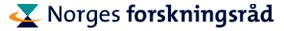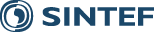Overview of Lectures

### Sunday to Tuesday: Prof. Per Christian Hansen

The lectures will give an introduction to linear inverse problems and their (classical) theory. This will by done by means of some simple linear problems, in the form of deconvolution problems. The lectures will start with some "toy problems" from geophysics and signal/image processing, and then use all the tool-of-the-trade from numerical linear algebra, primarily the SVD, to explain the inherent difficulties. With this background, you will be ready for the parameter-estimation problems discussed later in the week. The lectures will be based on the surver paper on deconvolution methods and Toeplitz matrices. However, the Toeplitz (computational) aspects will be toned down and the focus will be on the above-mentioned aspects.

### Tuesday to Thursday: Dr. Martin Burger

The lectures will focus on parameter identification problems for PDEs. Also these lectures will start with basic material, typical challenges, problem formulation (output least squares, etc), and thereafter move on to more complex methods and solution strategies (Lagrange multipliers, level set, software aspects, etc). Examples will be taken from material testing and geophysics, and the inverse conductivity problem will be mentioned briefly.

Lecture notes:

### Thursday: Prof. Xue-Cheng Tai

The lectures will discuss parameter identification by level set and related methods. We start with the phase filed model where one need to find interfaces for motion by mean curvature. Then some other interface problem like image segmentation and optimal shape design problems will be presented. After this, a relation between the phase field model and the level set method will be explained. In the end, some new piecewise constant level set methods will be presented and their applications, strengthens and weakness will be discussed. Some details shall be given for these new piecewise constant level set methods.

### Friday: Dr. Bjørn Fredrik Nielsen and Dr. Marius Lysaker

The lectures will focus on various inverse problems arising in connection with ECG (electrocardiogram) recordings. More specifically, the three lectures can roughly be described as follows:

1. A brief introduction to the Bidmain equations, modeling the electrical activity in the body, is given. We will present numerical experiments and discuss various numerical challenges.
2. This lecture will focus on the classical inverse problem of electrocardiography: Is it possible to compute the electrical potential at the surface of the heart from body surface measurements? This is an ill-posed linear problem, and it may be formulated in terms of an elliptic PDE. In the first part of the lecture, this challenge will be studied on an idealized geometry by applying Fourier analysis. Thereafter, the general case is treated and numerical experiments will be presented.
3. The purpose of the third lecture is to present a framework, developed by our research group at Simula Research Laboratory, suitable for identifying heart infarctions. This leads to an ill-posed nonlinear problem for a parabolic PDE. The potentially complex geometrical structures of infarctions will be represented by applying level set techniques.
Lecture notes:

Emphasis will be put on making the lectures as self-contained as possible, and we assume no specialized knowledge, except for a general knowledge of PDEs, numerical methods and analysis.

Published October 20, 2010